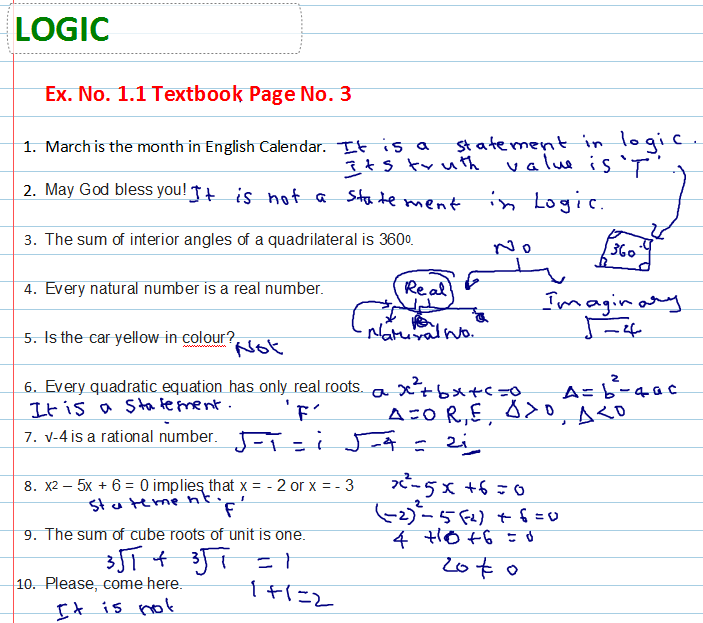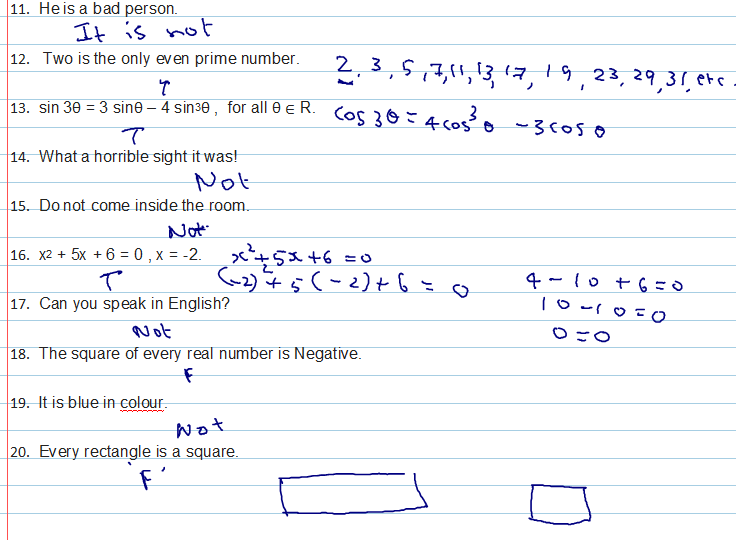9TH MAHARASHTRA: MATHS I 10TH TAMIL NADU: MATHS, SCIENCE, SSLC 10TH NEW TEXT BOOK PDF DOWNLOAD CBSE 10TH: MATHS 2019 BOARD PAPER SOLUTION: 2019 BOARD PAPER SOLUTION

Ex. No. 1.1

State which of the following sentences are statements. Justify your answer. In case of statements, Write down the truth value. [video]

1. March is the month in English Calendar.
2. May God bless you!
3. The sum of interior angles of a quadrilateral is 3600.
4. Every natural number is a real number.
5. Is the car yellow in colour?
6. Every quadratic equation has only real roots.
7. √-4 is a rational number.
8. x2 – 5x + 6 = 0 implies that x = - 2 or x = - 3
9. The sum of cube roots of unit is one.
11. He is a bad person.
12. Two is the only even prime number.
13. sin 3θ = 3 sinθ – 4 sin3θ ,  for all θ ∈ R.
14. What a horrible sight it was!
15. Do not come inside the room.
16. x2 + 5x + 6 = 0 , x = -2.
17. Can you speak in English?
18. The square of every real number is Negative.
19. It is blue in colour.
20. Every rectangle is a square.